x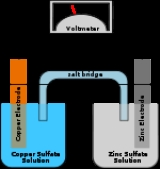Galvanic cellEncyclopedia
A Galvanic cell, or Voltaic cell, named after Luigi Galvani
Luigi Galvani
Luigi Aloisio Galvani was an Italian physician and physicist who lived and died in Bologna. In 1791, he discovered that the muscles of dead frogs legs twitched when struck by a spark...

, or Alessandro Volta
Alessandro Volta
Count Alessandro Giuseppe Antonio Anastasio Gerolamo Umberto Volta was a Lombard physicist known especially for the invention of the battery in 1800.-Early life and works:...

respectively, is an electrochemical cell
Electrochemical cell
An electrochemical cell is a device capable of either deriving electrical energy from chemical reactions, or facilitating chemical reactions through the introduction of electrical energy. A common example of an electrochemical cell is a standard 1.5-volt "battery"...

that derives electrical energy from spontaneous redox reaction taking place within the cell. It generally consists of two different metals connected by a salt bridge
Salt bridge
A salt bridge, in chemistry, is a laboratory device used to connect the oxidation and reduction half-cells of a galvanic cell , a type of electrochemical cell...

, or individual half-cells separated by a porous membrane.

Volta was the inventor of the voltaic pile
Voltaic pile
A voltaic pile is a set of individual Galvanic cells placed in series. The voltaic pile, invented by Alessandro Volta in 1800, was the first electric battery...

, the first electrical battery
Battery (electricity)
An electrical battery is one or more electrochemical cells that convert stored chemical energy into electrical energy. Since the invention of the first battery in 1800 by Alessandro Volta and especially since the technically improved Daniell cell in 1836, batteries have become a common power...

. In common usage, the word "battery" has come to include a single Galvanic cell, but a battery properly consists of multiple cells.

## History

In 1780, Luigi Galvani discovered that when two different metal
Metal
A metal , is an element, compound, or alloy that is a good conductor of both electricity and heat. Metals are usually malleable and shiny, that is they reflect most of incident light...

s (copper and zinc for example) were connected together and then both touched to two different parts of a nerve of a frog leg at the same time, they made the leg contract. He called this "animal electricity". The voltaic pile
Voltaic pile
A voltaic pile is a set of individual Galvanic cells placed in series. The voltaic pile, invented by Alessandro Volta in 1800, was the first electric battery...

invented by Alessandro Volta
Alessandro Volta
Count Alessandro Giuseppe Antonio Anastasio Gerolamo Umberto Volta was a Lombard physicist known especially for the invention of the battery in 1800.-Early life and works:...

in the 1800s is similar to the galvanic cell. These discoveries paved the way for electrical batteries
Battery (electricity)
An electrical battery is one or more electrochemical cells that convert stored chemical energy into electrical energy. Since the invention of the first battery in 1800 by Alessandro Volta and especially since the technically improved Daniell cell in 1836, batteries have become a common power...

. Volta's cell was named an IEEE Milestone in 1999.

## Description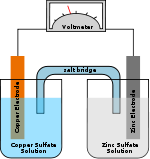A Galvanic cell consists of two half-cells. In its simplest form, each half-cell consists of a metal and a solution of a salt of the metal. The salt solution contains a cation of the metal and an anion to balance the charge on the cation. In essence, the half-cell contains the metal in two oxidation state
Oxidation state
In chemistry, the oxidation state is an indicator of the degree of oxidation of an atom in a chemical compound. The formal oxidation state is the hypothetical charge that an atom would have if all bonds to atoms of different elements were 100% ionic. Oxidation states are typically represented by...

s and the chemical reaction in the half-cell is an oxidation-reduction
Redox
Redox reactions describe all chemical reactions in which atoms have their oxidation state changed....

(redox) reaction, written symbolically in reduction direction as
Mn+ (oxidized species) + n M (reduced species)

In a galvanic cell one metal is able to reduce the cation of the other and, conversely, the other cation can oxidize the first metal. The two half-cells must be physically separated so that the solutions do not mix together. A salt bridge
Salt bridge
A salt bridge, in chemistry, is a laboratory device used to connect the oxidation and reduction half-cells of a galvanic cell , a type of electrochemical cell...

or porous plate is used to separate the two solutions yet keep the respective charges of the solutions from separating, which would stop the chemical reactions.

The number of electrons transferred in both directions must be the same, so the two half-cells are combined to give the whole-cell electrochemical reaction. For two metals A and B:
An+ + n A
Bm+ + m B
m A + n Bm+ n B + m An+

This is not the full working, as anions must also be transferred from one half-cell to the other. When a metal in one half-cell is oxidized, anions must be transferred into that half-cell to balance the electrical charge of the cation produced. The anions are released from the other half-cell where a cation is reduced to the metallic state. Thus, the salt bridge or porous membrane serves both to keep the solutions apart and to allow the flow of anions in the direction opposite to the flow of electrons in the wire connecting the electrodes.

The voltage of the Galvanic cell is the sum of the voltages of the two half-cells. It is measured by connecting a voltmeter
Voltmeter
A voltmeter is an instrument used for measuring electrical potential difference between two points in an electric circuit. Analog voltmeters move a pointer across a scale in proportion to the voltage of the circuit; digital voltmeters give a numerical display of voltage by use of an analog to...

to the two electrodes. The voltmeter has very high resistance
Electrical resistance
The electrical resistance of an electrical element is the opposition to the passage of an electric current through that element; the inverse quantity is electrical conductance, the ease at which an electric current passes. Electrical resistance shares some conceptual parallels with the mechanical...

, so the current flow is effectively negligible. When a device such as an electric motor is attached to the electrodes, a current flows and redox reactions occur in both half-cells. This will continue until the concentration of the cations that are being reduced goes to zero.

For the Daniell cell
Daniell cell
The Daniell cell was invented in 1836 by John Frederic Daniell, a British chemist and meteorologist, and consisted of a copper pot filled with a copper sulfate solution, in which was immersed an unglazed earthenware container filled with sulfuric acid and a zinc electrode...

, depicted in the figure, the two metals are zinc
Zinc
Zinc , or spelter , is a metallic chemical element; it has the symbol Zn and atomic number 30. It is the first element in group 12 of the periodic table. Zinc is, in some respects, chemically similar to magnesium, because its ion is of similar size and its only common oxidation state is +2...

and copper
Copper
Copper is a chemical element with the symbol Cu and atomic number 29. It is a ductile metal with very high thermal and electrical conductivity. Pure copper is soft and malleable; an exposed surface has a reddish-orange tarnish...

and the two salts are sulfate
Sulfate
In inorganic chemistry, a sulfate is a salt of sulfuric acid.-Chemical properties:...

s of the respective metal. Zinc is the oxidized metal so when a device is connected to the electrodes, the electrochemical reaction is
Zn + → + Cu

The zinc electrode is dissolved and copper is deposited on the copper electrode (as copper ions become reduced to copper metal). By definition, the cathode
Cathode
A cathode is an electrode through which electric current flows out of a polarized electrical device. Mnemonic: CCD .Cathode polarity is not always negative...

is the electrode where reduction (gain of electrons) takes place, so the copper electrode is the cathode. The cathode attracts cations, so has a negative charge when current is discharging. In this case, copper is the cathode and zinc the anode.

Galvanic cells are typically used as a source of electrical power. By their nature they produce direct current
Direct current
Direct current is the unidirectional flow of electric charge. Direct current is produced by such sources as batteries, thermocouples, solar cells, and commutator-type electric machines of the dynamo type. Direct current may flow in a conductor such as a wire, but can also flow through...

. For example, a lead-acid battery
Lead–acid batteries, invented in 1859 by French physicist Gaston Planté, are the oldest type of rechargeable battery. Despite having a very low energy-to-weight ratio and a low energy-to-volume ratio, their ability to supply high surge currents means that the cells maintain a relatively large...

contains a number of galvanic cells. The two electrodes are effectively lead and lead oxide.

The Weston cell
Weston cell
The Weston cell, invented by Edward Weston in 1893, is a wet-chemical cell that produces a highly stable voltage suitable as a laboratory standard for calibration of voltmeters...

was adopted as an International Standard for voltage in 1911. The anode is a cadmium
Cadmium is a chemical element with the symbol Cd and atomic number 48. This soft, bluish-white metal is chemically similar to the two other stable metals in group 12, zinc and mercury. Similar to zinc, it prefers oxidation state +2 in most of its compounds and similar to mercury it shows a low...

mercury
Mercury (element)
Mercury is a chemical element with the symbol Hg and atomic number 80. It is also known as quicksilver or hydrargyrum...

amalgam
Amalgam (chemistry)
An amalgam is a substance formed by the reaction of mercury with another metal. Almost all metals can form amalgams with mercury, notable exceptions being iron and platinum. Silver-mercury amalgams are important in dentistry, and gold-mercury amalgam is used in the extraction of gold from ore.The...

, the cathode is made of pure mercury, the electrolyte is a (saturated) solution of cadmium sulfate
Cadmium sulfate is the name of a series of related inorganic compounds with the formula CdSO4.xH2O. The most common form is the monohydrate CdSO4.H2O, but two other forms are known CdSO4.8/3H2O and the anhydrous salt...

and the depolarizer is a paste of mercurous sulfate. When the electrolyte solution is saturated the voltage of the cell is very reproducible, hence its use as a standard.

## Cell voltage

The standard electrical potential of a cell can be determined by use of a standard potential table for the two half cell
Half cell
A half-cell is a structure that contains a conductive electrode and a surrounding conductive electrolyte separated by a naturally occurring Helmholtz double layer. Chemical reactions within this layer momentarily pump electric charges between the electrode and the electrolyte, resulting in a...

s involved. The first step is to identify the two metals reacting in the cell. Then one looks up the standard electrode potential
Standard electrode potential
In electrochemistry, the standard electrode potential, abbreviated E° or E , is the measure of individual potential of a reversible electrode at standard state, which is with solutes at an effective concentration of 1 mol dm−3, and gases at a pressure of 1 atm...

,
E0, in volt
Volt
The volt is the SI derived unit for electric potential, electric potential difference, and electromotive force. The volt is named in honor of the Italian physicist Alessandro Volta , who invented the voltaic pile, possibly the first chemical battery.- Definition :A single volt is defined as the...

s, for each of the two half reactions
Half-reaction
A half reaction is either the oxidation or reduction reaction component of a redox reaction. A half reaction is obtained by considering the change in oxidation states of individual substances involved in the redox reaction.-Example:...

. The standard potential for the cell is equal to the more positive E0 value minus the more negative E0 value.

For example, in the figure above the solutions are CuSO4 and ZnSO4. Each solution has a corresponding metal strip in it, and a salt bridge
Salt bridge
A salt bridge, in chemistry, is a laboratory device used to connect the oxidation and reduction half-cells of a galvanic cell , a type of electrochemical cell...

or porous disk connecting the two solutions and allowing SO42− ions to flow freely between the copper and zinc solutions. In order to calculate the standard potential one looks up copper and zinc's half reactions and finds:
Cu2+ + 2 Cu: E0 = +0.34 V
Zn2+ + 2 Zn: E0 = −0.76 V

Thus the overall reaction is:
Cu2+ + Zn Cu + Zn2+

The standard potential for the reaction is then +0.34 V − (−0.76 V) = 1.10 V. The polarity of the cell is determined as follows. Zinc metal is more strongly reducing than copper metal as shown by the fact that the standard (reduction) potential for zinc is more negative than that of copper. Thus, zinc metal will lose electrons to copper ions and develop a positive electrical charge. The equilibrium constant, K, for the cell is given by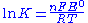where F is the Faraday constant, R is the gas constant
Gas constant
The gas constant is a physical constant which is featured in many fundamental equations in the physical sciences, such as the ideal gas law and the Nernst equation. It is equivalent to the Boltzmann constant, but expressed in units of energy The gas constant (also known as the molar, universal,...

and T is the temperature in kelvin
Kelvin
The kelvin is a unit of measurement for temperature. It is one of the seven base units in the International System of Units and is assigned the unit symbol K. The Kelvin scale is an absolute, thermodynamic temperature scale using as its null point absolute zero, the temperature at which all...

s. For the Daniell cell K is approximately equal to 1.5×1037. Thus, at equilibrium, a few electrons are transferred, enough to cause the electrodes to be charged.

Actual half-cell potentials must be calculated by using the Nernst equation
Nernst equation
In electrochemistry, the Nernst equation is an equation that can be used to determine the equilibrium reduction potential of a half-cell in an electrochemical cell. It can also be used to determine the total voltage for a full electrochemical cell...

as the solutes are unlikely to be in their standard states,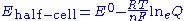where Q is the reaction quotient
Reaction quotient
In chemistry, a reaction quotient: Qr is a function of the activities or concentrations of the chemical species involved in a chemical reaction. In the special case that the reaction is at equilibrium the reaction quotient is equal to the equilibrium constant....

. This simplifies to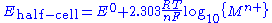where {Mn+} is the activity
Activity (chemistry)
In chemical thermodynamics, activity is a measure of the “effective concentration” of a species in a mixture, meaning that the species' chemical potential depends on the activity of a real solution in the same way that it would depend on concentration for an ideal solution.By convention, activity...

of the metal ion in solution. The metal electrode is in its standard state so by definition has unit activity. In practice concentration is used in place of activity. The potential of the whole cell is obtained by combining the potentials for the two half-cells, so it depends on the concentrations of both dissolved metal ions.

The value of 2.303R/F is 0.19845×10−3 V/K, so at 25 °C (298.15 K) the half-cell potential will change by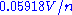if the concentration of a metal ion is increased or decreased by a factor of 10.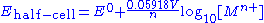These calculations are based on the assumption that all chemical reactions are in equilibrium
Chemical equilibrium
In a chemical reaction, chemical equilibrium is the state in which the concentrations of the reactants and products have not yet changed with time. It occurs only in reversible reactions, and not in irreversible reactions. Usually, this state results when the forward reaction proceeds at the same...

. When a current flows in the circuit, equilibrium conditions are not achieved and the cell potential will usually be reduced by various mechanisms, such as the development of overpotential
Overpotential
Overpotential is an electrochemical term which refers to the potential difference between a half-reaction's thermodynamically determined reduction potential and the potential at which the redox event is experimentally observed. The term is directly related to a cell's voltage efficiency...

s. Also, since chemical reactions occur when the cell is producing power, the electrolyte concentrations change and the cell voltage is reduced. A consequence of the temperature dependency of standard potentials is that the voltage produced by a galvanic cell is also temperature dependent.

## Galvanic corrosion

Galvanic corrosion is a process that degrades metals electrochemically.
This corrosion
Corrosion
Corrosion is the disintegration of an engineered material into its constituent atoms due to chemical reactions with its surroundings. In the most common use of the word, this means electrochemical oxidation of metals in reaction with an oxidant such as oxygen...

occurs when two dissimilar metals are placed in contact with each other in the presence of an electrolyte
Electrolyte
In chemistry, an electrolyte is any substance containing free ions that make the substance electrically conductive. The most typical electrolyte is an ionic solution, but molten electrolytes and solid electrolytes are also possible....

, such as salt water, forming a galvanic cell. A cell can also be formed if the same metal is exposed to two different concentrations of electrolyte. The resulting electrochemical potential then develops an electric current that electrolytically dissolves the less noble material.

## Cell types

• Concentration cell
Concentration cell
A concentration cell is a limited form of a galvanic cell that has two equivalent half-cells of the same material differing only in concentrations. One can calculate the potential developed by such a cell using the Nernst Equation. A concentration cell produces a voltage as it attempts to reach...

• Electrolytic cell
Electrolytic cell
An electrolytic cell decomposes chemical compounds by means of electrical energy, in a process called electrolysis; the Greek word lysis means to break up. The result is that the chemical energy is increased...

• Electrochemical cell
Electrochemical cell
An electrochemical cell is a device capable of either deriving electrical energy from chemical reactions, or facilitating chemical reactions through the introduction of electrical energy. A common example of an electrochemical cell is a standard 1.5-volt "battery"...

• Lemon battery
Lemon battery
A lemon battery is a device used in experiments proposed in many science textbooks around the world. It is made by inserting two different metallic objects, for example a galvanized nail and a copper coin, into a lemon. The copper coin serves as the positive electrode or cathode and the galvanized...

• Alessandro Volta
Alessandro Volta
Count Alessandro Giuseppe Antonio Anastasio Gerolamo Umberto Volta was a Lombard physicist known especially for the invention of the battery in 1800.-Early life and works:...

• Battery (electricity)
Battery (electricity)
An electrical battery is one or more electrochemical cells that convert stored chemical energy into electrical energy. Since the invention of the first battery in 1800 by Alessandro Volta and especially since the technically improved Daniell cell in 1836, batteries have become a common power...

• Bio-nano generator
• Desulfation
Desulfation
Desulfation is the process of reversing the process of sulfation that occurs to a lead-acid battery over time. Desulfation restores, at least partially, the ability of the battery to hold a charge over the life of the battery originally caused by sulfation). Sulfation is the formation of large...

• Electrode potential
Electrode potential
Electrode potential, E, in electrochemistry, according to an IUPAC definition, is the electromotive force of a cell built of two electrodes:* on the left-hand side is the standard hydrogen electrode, and...

• Electrosynthesis
Electrosynthesis
Electrosynthesis in organic chemistry is the synthesis of chemical compounds in a electrochemical cell The main advantage of electrosynthesis over an ordinary redox reaction is avoidance of the potential wasteful other half-reaction and the ability to precisely tune the required potential...

• Isotope electrochemistry
Isotope electrochemistry
Isotope electrochemistry is a field within electrochemistry concerned with various topics like electrochemical separation of isotopes, electrochemical estimation of isotopic exchange equilibrium constants , electrochemical kinetic isotope effect, electrochemical isotope sensors, etc.It is an active...

• Bioelectrochemical reactor
Bioelectrochemical reactor
Bioelectrochemical reactors are a type of bioreactor where bioelectrochemical processes can take place. They are used in bioelectrochemical syntheses, environmental remediation and electrochemical energy conversion...

• Enzymatic biofuel cell
• Electrohydrogenesis
Electrohydrogenesis
Electrohydrogenesis or biocatalyzed electrolysis is the name given to a process for generating hydrogen gas from organic matter being decomposed by bacteria. This process uses a modified fuel cell to contain the organic matter and water...

• Electrochemical engineering
Electrochemical engineering
Electrochemical engineering is the branch of engineering dealing with the technological applications of electrochemical phenomena...

• Galvanic series
Galvanic series
The galvanic series determines the nobility of metals and semi-metals. When two metals are submerged in an electrolyte, while electrically connected, the less noble will experience galvanic corrosion. The rate of corrosion is determined by the electrolyte and the difference in nobility...

• Sacrificial anode
• Salt bridge
Salt bridge
A salt bridge, in chemistry, is a laboratory device used to connect the oxidation and reduction half-cells of a galvanic cell , a type of electrochemical cell...

• Volt
Volt
The volt is the SI derived unit for electric potential, electric potential difference, and electromotive force. The volt is named in honor of the Italian physicist Alessandro Volta , who invented the voltaic pile, possibly the first chemical battery.- Definition :A single volt is defined as the...

• Voltaic pile
Voltaic pile
A voltaic pile is a set of individual Galvanic cells placed in series. The voltaic pile, invented by Alessandro Volta in 1800, was the first electric battery...